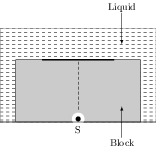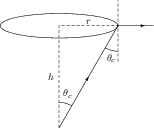# Total Internal Reflection

## Problems from IIT JEE

Problem (IIT JEE 2014):A point source S is placed at the bottom of a transparent block of height 10 m and refractive index 2.72. It is immersed in a lower refractive index liquid as shown in the figure. It is found that the light emerging from the block to the liquid forms a circular bright spot of diameter 11.54 mm on the top of the block. The refractive index of the liquid is,

1. 1.21
2. 1.30
3. 1.36
4. 1.42

Solution:The rays stop coming to liquid side if the angle of incidence at the block-liquid interface is greater than the critical angle $\theta_c$ (see figure). Let $\mu_b=2.72$ be the refractive index of the block and $\mu_l$ be the refractive index of the liquid. The critical angle is given by, \begin{align} \label{pxb:eqn:1} \sin\theta_c={\mu_l}/{\mu_b}. \end{align} From geometry, \begin{align} \label{pxb:eqn:2} \sin\theta_c={r}/{\sqrt{r^2+h^2}}. \end{align} Solve above equations to get, \begin{align} \mu_l=\frac{\mu_b r}{\sqrt{r^2+h^2}}=\frac{2.72(11.54/2)}{\sqrt{(11.54/2)^2+10^2}}=1.36.\nonumber \end{align}